U.S. Department of Transportation
1200 New Jersey Avenue, SE
Washington, DC 20590
202-366-4000

Federal Highway Administration Research and Technology
Coordinating, Developing, and Delivering Highway Transportation InnovationsThis report is an archived publication and may contain dated technical, contact, and link information
 Federal Highway Administration > Publications > Research Publications > 05081 > Design of Continuously Reinforced Concrete Pavements Using Glass Fiber Reinforced Polymer Rebars
 Publication Number: FHWA-HRT-05-081 Date: October 2005

# Chapter 3. Analytical Study

To estimate the development of shrinkage and thermal stresses in a freely supported reinforced concrete slab, a representative concrete prismatic model containing a longitudinal reinforcing rebar at its center with width (or reinforcing space in CRCP) B, height (or thickness in CRCP) H, length L, and rebar diameter 2rr is considered. Then, for simplification of analysis, the model is modified into an equivalent cylinder with the corresponding equivalent diameter 2R accompanied by the same length and rebar diameter as those for the prismatic model. Schematic details of the models are shown in figures 5 and 6.

Figure 4. Equation. R.

 R = HB π

Figure 5. Drawing. Schematic details of representative reinforced concrete prism.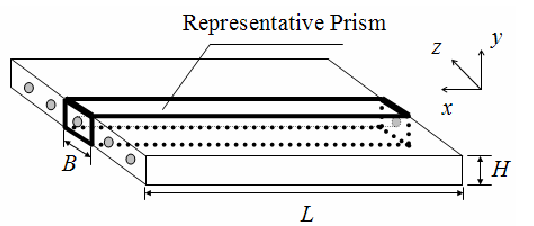Figure 6. Drawing. Schematic details of equivalent cylinder used in analyses.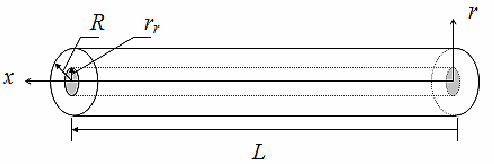Adopting the shear-lag theory, there are several assumptions applied to this analysis: (1) the concrete and reinforcement exhibit elastic behavior, (2) a perfect bond between concrete and reinforcement exists at an infinitely thin interface, (3) the stiffnesses of the concrete and the reinforcement in the radial (r-) direction are the same, (4) the strain in the concrete εc at a distance R from the x-axis is equivalent to the restraint-free concrete strain due to the shrinkage or temperature variation, and (5) the temperature distribution in the concrete and reinforcement is uniform in the radial direction.(27) The effects of concrete's radial shrinkage on concrete stress development in the longitudinal (x-) direction are neglected. Also, the effects from the CTE discrepancies between concrete and reinforcement in the radial direction are neglected.

When the concrete is subjected to a strain in the longitudinal (x-) direction,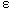c, the rate of transfer of load from concrete to reinforcement can be assumed as:

Figure 7. Equation. dP/dx.

 dP = Co ( u - v ) dx

where P = load in the reinforcement; Co = a constant; and v and u = axial displacements at r = R and r = rr , respectively. It is also known from force equilibrium that dP/dx = 2πrτ, where τ is the axial shear stress varying along the radial direction. This gives:

Figure 8. Equation.t(r).

 τ ( r ) = dP 1 dx 2 π r

The relative displacement between u and v can be obtained by:

Figure 9. Equation. (u-v) in the form of integrating γ(r).

 ( u - v ) = ∫ R γ ( r ) dr = ∫ R τ ( r ) dr rr rr Gc

where γ(r) = axial shear strain varying along the radial direction; and Gc = shear modulus of concrete. By solving figure 9, one then gets:

Figure 10. Equation. (u-v) in the solved form.

 ( u - v ) = dP ln ( R / rr ) dx 2 π Gc

Hence,

Figure 11. Equation.Co.

 Co = 2 π Gc ln ( R / rr )

Also, dv/dx = εc and du/dx = εr. Therefore,

Figure 12. Equation. d2P/dx2.

 d2P = Co ( εr - εc ) dx2

The restraint-free concrete axial strain, εc, in the above equation can be substituted with either shrinkage strain at any time t (in days (d)), εc,s(t), or thermal strain, εc,t, which are given by:

Figure 13. Equation. εc,s ( t ).

 εc,s ( t ) = -t ( εc,s )ult 35 + t

and

Figure 14. Equation. εc,t.

εc,t = Δ T αt

where (εc,s)ult = ultimate shrinkage strain for drying at 40 percent RH; εT = temperature variation; and ac = CTE of concrete. εc,s(t) is an empirical equation for moist cured concrete.(28) The reinforcement axial strains due to concrete shrinkage, εr,s, or due to temperature variation, εr,t, are shown as:

Figure 15. Equation. εr,s.

 εr,s = P ArEr

and

Figure 16. Equation. εr,t.

 εr,t = Δ T α + P ArEr

where Ar = reinforcement cross-sectional area; Er = Young's modulus of the reinforcement; and ar = CTE of the reinforcement.

Substituting figures 13-16 into figure 12 gives the governing differential equation for P, and then by solving the differential equation with the boundary conditions that P = 0 at x = 0 and x = L, the reinforcement force, P(x), can be obtained. The axial force equilibrium with the average axial concrete stress, (sc)avg, must also be satisfied at any x location. In other words, P + (sc)avg Ac = 0, where Ac is the concrete cross-sectional area. Hence, the average axial concrete stress can be given as follows:

Figure 17. Equation. ( σc,s )avg.

 ( σc,s )avg = ρ Er t ( εc,s )ult 1 - cosh β L - x 2 35 + t cosh β L 2

and

Figure 18. Equation. ( σc,t )avg.

 ( σc,t )avg = - ρ Er Δ T ( αc - αr ) 1 - cosh β L - x 2 cosh β L 2

where ( σc,s )avg, ( σc,t )avg and (sc,t)avg = average axial concrete stresses induced by concrete shrinkage and temperature variation, respectively; r = reinforcing ratio (Ar/Ac); and

Figure 19. Equation. β.

 β = 2 Gc / Er r2r ln ( R / rr )

The negative values from figures 17 and 18 indicate compressive axial stresses in the concrete. The maximum axial stress in concrete can be simply found at the midpoint, x = L / 2.

### Material Parameters for Analytical Approximation

In addition to the concrete shrinkage strain, εc,s(t), shown in figure 13, the Young's modulus of concrete, Ec, is also employed as the time-dependent parameter in the shrinkage stress analysis. This leads the shrinkage stress analysis to be dependent on the elapsed time, t(d). The time-dependent Young's modulus of concrete can be evaluated by:

Figure 20. Equation. Ec(t).

Ec ( t ) = Ec,28 [ 0.52 + 0.15 ln ( t ) ] for t ≤ 28

and

Figure 21. Equation. Ec.

Ec = 1.019 Ec,28 for t > 28

where Ec,28 = Young's modulus of concrete at 28 d.(29) In the thermal stress analysis, the concrete stresses at 28 d are estimated for different temperature variations; Ec,28 was therefore employed for the Young's modulus of concrete in the analysis. Table 1 lists a set of model parameters and material properties used in the analytical study. The analytical model with a width of B = 15.24 cm (6 inches) and a height of H = 25.4 cm (10 inches) was examined in the study. Shown in the table are the Young's moduli and CTEs of two different concretes whose types of coarse aggregate used are granite and siliceous river gravel, respectively. (30) In addition, the GFRP rebar used in the analyses is assumed to be C-BAR® deformed FRP rebar, manufactured by Marshall Industry Composite, Inc.

### Analytical Results and Discussion

In figure 22, the maximum average tensile stresses in the concrete due to concrete shrinkage are calculated over a period of time; the stresses induced by steel and GFRP reinforcements are shown in the figure for the purpose of comparison, and the granite aggregate concrete is used in the shrinkage stress analysis. In the calculation, number 5 rebars with radii of 7.94 mm (0.3125 inch; r = 0.00519) are employed for the models having a length of L = 1.524 m (60 inches (5 ft)). The figure reveals that the maximum concrete stress level created by GFRP rebar is about one-fifth of that created by steel rebar, which is a ratio about the same as that of the longitudinal GFRP rebar's elastic modulus to the steel rebar's elastic modulus. The lower stress level by GFRP rebar results from the GFRP's lower elastic modulus providing the concrete with less restraint while the concrete shrinks.

Table 1. Model parameters and material properties used in analytical approximation.
Parameter and PropertiesValue
Width, B (cm (inches))15.24 (6.0)
Height, H (cm (inches))25.40 (10.0)
Length, L (m (inches))1.52 (60.0)
Ultimate concrete shrinkage strain, (ec,s)ult (μ ε)800.00
Young's modulus of concrete at 28 d, Ec,28 (GPa (x 106 lbf/in2))33.10 (4.80)1 and 34.48 (5.0)2
Poisson's ratio of concrete, nc0.20
Young's modulus of steel rebar (GPa (x 106 lbf/in2))200.00 (29.00)
Longitudinal Young's modulus of GFRP rebar (GPa (x 106 lbf/in2))40.00 (5.80)
CTE of concrete, ac ( μ ε /oC ( μ ε / °F) )10.26 (5.7)1 and 14.40 (8.0)2
CTE of steel rebar, ar,s ( μ ε /oC ( μ ε / °F) )11.88 (6.6)
CTE of GFRP rebar, ar,g ( μ ε /oC ( μ ε / °F) )9.36(5.2)

1Granite coarse aggregate.
2Siliceous river gravel coarse aggregate used.

1 megapoundforce per square inch (106 lbf/in2) = 6.89 gigapascal (GPa)
1 kilopoundforce per square inch (ksi) = 6.89 megapascal (MPa)

Figure 22. Graph. Maximum average tensile stress in concrete versus time (ρ = 0.00519 and L = 1.524 m (60 inches)).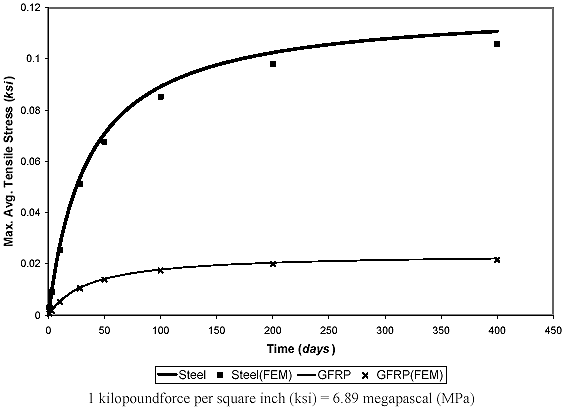The cases for different sizes of GFRP rebars (numbers 3 through 6 (r = 0.00184 through 0.00739)) are presented in figure 23, showing that the maximum concrete stress increases with an increase in the reinforcement ratio. This is because of the greater restraint provided to the concrete shrinkage by a higher reinforcement ratio. The maximum concrete stresses for different slab lengths, L, are examined in figure 24. The stresses increase with increases in length, L, increasingly converging to ρErεc,s(t) when L approaches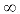. With the set of parameters (table 1) used, the maximum concrete stress already converges to ρErεc,s(t) reaches about 0.305 m (12 inches).

The discrepancy in CTEs between the concrete and reinforcement causes thermal stresses in both the concrete and reinforcement as temperatures change. Figures 23 and 24 show the plots of the maximum average axial stress in concrete versus temperature increase (positive εT) for two different concrete CTEs. The CTE of granite aggregate concrete is lower than that of steel rebar and higher than that of GFRP rebar (table 1). As shown in figure 25, this CTE of concrete causes tensile concrete stress when using steel rebar and compressive concrete stress when using GFRP rebar as temperature increases; in the figure, the positive value represents the tensile stress, and the negative value the compressive stress.

Figure 23. Graph. Maximum average tensile stress in concrete versus time
(L = 1.524 m (60 inches)) and different GFRP reinforcing ratios, ρ).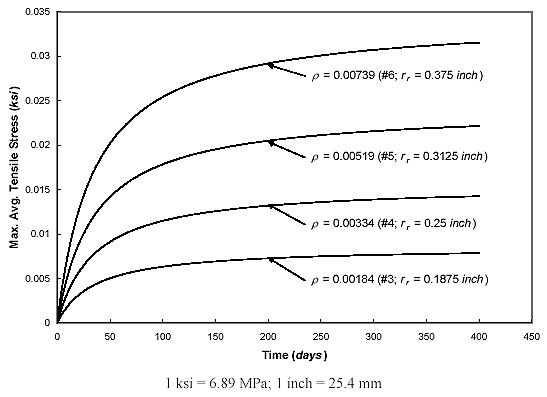Figure 24. Graph. Maximum average tensile stress in concrete versus time
(ρ = 0.00519 GFRP and different slab lengths, L).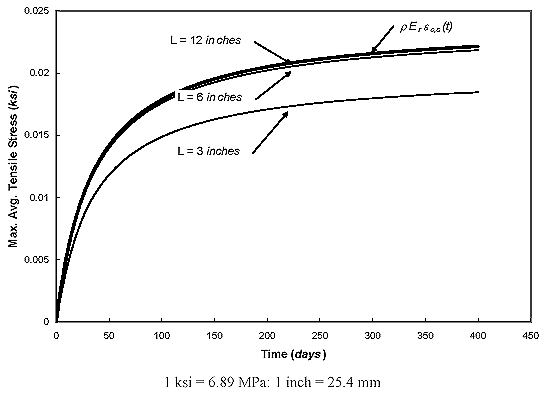Figure 25. Graph. Maximum average axial stress in concrete versus temperature change (r = 0.00519 and ρ = 1.524 m (60 inches)).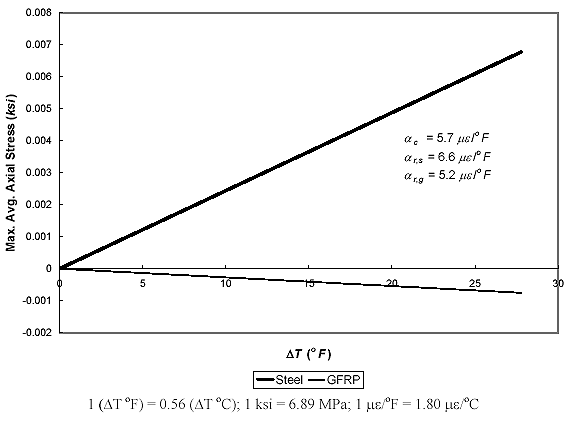The siliceous river gravel aggregate concrete has a CTE higher than both CTEs of steel and GFRP rebars, and temperature increases lead to compressive stress development in the concrete for both reinforcement cases, as shown in figure 26. These compressive and tensile states are reversed as temperature decreases (negative εT). As can be seen in both figures 25 and 26, the absolute values of thermal concrete stresses from GFRP rebar are less than those from steel rebar, mainly because of GFRP rebar's lower elastic modulus. In addition, both the magnitude and sign of the stress caused by temperature variation can be controlled by using different combinations of concrete and reinforcement CTEs.

Figure 26. Graph. Maximum average axial stress in concrete versus temperature change (ρ = 0.00519 and L = 1.524 m (60 inches)).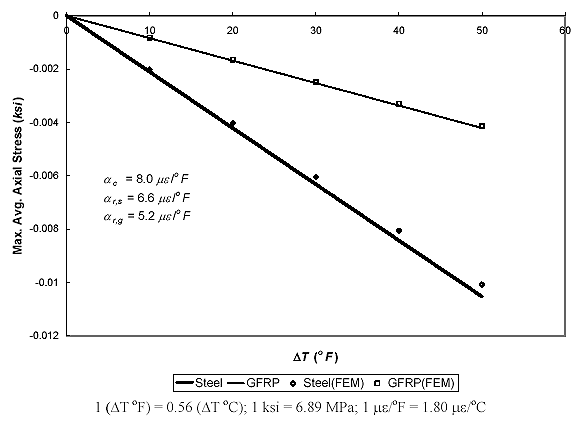#### Comparison with Finite Element Model

Using the FE analysis program, ABAQUS, a finite element model (FEM) of a freely supported, reinforced concrete slab has been created, and the results from the shrinkage and thermal analytical models are compared with the FEM results. The concrete slab is modeled as four-node, 2-D elements under plane stress conditions, and the reinforcement is modeled as beam elements with circular cross sections. The thickness of the concrete 2-D plane stress elements is the width, B, of the concrete prismatic model. The bond-slip between concrete and reinforcement is modeled by using horizontal spring elements, and the bond is assumed to be perfect. All of the material properties are assumed to be the same as those used in the analytical model (table 1); in this study, the spring stiffnesses used to simulate the perfect bond, which depend on Young's modulus of concrete, are 1.303 GN/m (7,443 kip/inch) and 1.251 GN/m (7,145 kip/inch) for granite concrete and siliceous river gravel concrete, respectively. The comparison (figures 20 and 24) between the analytical and FE methods shows a good agreement of the maximum average axial stresses in the concrete.

The axial concrete stress distribution along the longitudinal (x-) direction for a GFRP-reinforced slab with a temperature variation of -27.8 °C (-50 °F) has also been calculated using both analytical and FE methods (figure 27).

Figure 27. Graph. Axial stress in concrete versus longitudinal location (comparison between analytical and FEM results).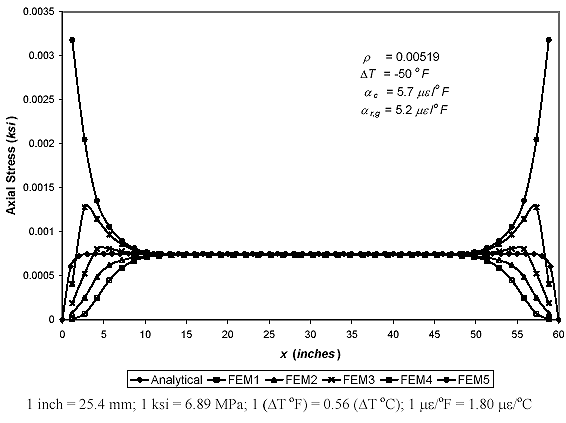Figure 27 shows the concrete stresses located at five different longitudinal layers of concrete. The layers are laterally located from the concrete surface to the interface between the concrete and reinforcement, and are represented by using the nomenclature of FEM1 through FEM5 in the figure; FEM1 represents the surface layer, and FEM5 represents the layer closest to the reinforcement. The results show that the axial stresses from the analytical model, which are averaged in the radial (r-) direction, agree well with the axial stresses from all the layers of the concrete FE element as the x-location approaches the middle of the section. From the FE results, it can be seen that the axial stresses are varied through the depth around the ends of the slab. This is simply caused by the fact that, along the depth direction, the more drastic change in axial displacement occurs around the ends rather than around the middle section due to the restraint from the reinforcement. The comparison also shows that the analytical solution of average axial concrete stresses (figure 18) is valid throughout the longitudinal (x-) direction, especially in the vicinity of the slab's middle section, proving the validity of the maximum average axial stresses in concrete at x = L/2 as previously shown.